# Rotation (mathematics)

﻿
Rotation (mathematics)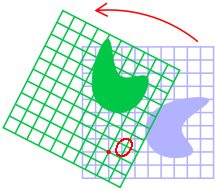Rotation of an object in two dimensions around a point O.

In geometry and linear algebra, a rotation is a transformation in a plane or in space that describes the motion of a rigid body around a fixed point. A rotation is different from a translation, which has no fixed points, and from a reflection, which "flips" the bodies it is transforming. A rotation and the above-mentioned transformations are isometries; they leave the distance between any two points unchanged after the transformation.

It is important to know the frame of reference when considering rotations, as all rotations are described relative to a particular frame of reference. In general for any orthogonal transformation on a body in a coordinate system there is an inverse transformation which if applied to the frame of reference results in the body being at the same coordinates. For example in two dimensions rotating a body clockwise about a point keeping the axes fixed is equivalent to rotating the axes counterclockwise about the same point while the body is kept fixed.

## Two dimensions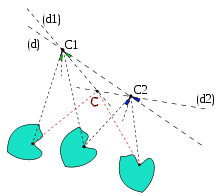A plane rotation around a point followed by another rotation around a different point results in a total motion which is either a rotation (as in this picture), or a translation.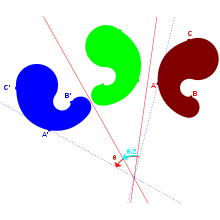A reflection against an axis followed by a reflection against a second axis not parallel to the first one results in a total motion that is a rotation around the point of intersection of the axes.

Only a single angle is needed to specify a rotation in two dimensions – the angle of rotation. To calculate the rotation two methods can be used, either matrix algebra or complex numbers. In each the rotation is acting to rotate an object counterclockwise through an angle θ about the origin.

### Matrix algebra

To carry out a rotation using matrices the point (x, y) to be rotated is written as a vector, then multiplied by a matrix calculated from the angle, θ, like so:$\begin{bmatrix} x' \\ y' \end{bmatrix} = \begin{bmatrix} \cos \theta & -\sin \theta \\ \sin \theta & \cos \theta \end{bmatrix} \begin{bmatrix} x \\ y \end{bmatrix}$.

where (x′, y′) are the co-ordinates of the point after rotation, and the formulae for x′ and y′ can be seen to be\begin{align} x'&=x\cos\theta-y\sin\theta\\ y'&=x\sin\theta+y\cos\theta. \end{align}

The vectors$\begin{bmatrix} x \\ y \end{bmatrix}$ and$\begin{bmatrix} x' \\ y' \end{bmatrix}$ have the same magnitude and are separated by an angle θ as expected.

### Complex numbers

Points can also be rotated using complex numbers, as the set of all such numbers, the complex plane, is geometrically a two dimensional plane. The point (x, y) in the plane is represented by the complex number$z = x + iy \,$

This can be rotated through an angle θ by multiplying it by e, then expanding the product using Euler's formula as follows:\begin{align} e^{i \theta} z &= (\cos \theta + i \sin \theta) (x + i y) \\ &= (x \cos \theta + i y \cos \theta + i x \sin \theta - y \sin \theta) \\ &= (x \cos \theta - y \sin \theta) + i (x \sin \theta + y \cos \theta) \\ &= x' + i y' , \end{align}

which gives the same result as before,\begin{align} x'&=x\cos\theta-y\sin\theta\\ y'&=x\sin\theta+y\cos\theta. \end{align}

Like complex numbers rotations in two dimensions are commutative, unlike in higher dimensions. They have only one degree of freedom, as such rotations are entirely determined by the angle of rotation.

## Three dimensions

Rotations in ordinary three-dimensional space differ from those in two dimensions in a number of important ways. Rotations in three dimensions are generally not commutative, so the order in which rotations are applied is important. They have three degrees of freedom, the same as the number of dimensions.

A three dimensional rotation can be specified in a number of ways. The most usual methods are as follows.

### Matrix algebra

As in two dimensions a matrix can be used to rotate a point (x, y, z) to a point (x′, y′, z′). The matrix used is a 3 × 3 matrix,$\mathbf{A} = \begin{pmatrix} a & b & c \\ d & e & f \\ g & h & i \end{pmatrix}$

This is multiplied by a vector representing the point to give the result$\mathbf{A} \begin{pmatrix} x \\ y \\ z \end{pmatrix} = \begin{pmatrix} a & b & c \\ d & e & f \\ g & h & i \end{pmatrix} \begin{pmatrix} x \\ y \\ z \end{pmatrix} = \begin{pmatrix} x' \\ y' \\ z' \end{pmatrix}$

The matrix A is a member of the three dimensional special orthogonal group, SO(3), that is it is an orthogonal matrix with determinant 1. That it is an orthogonal matrix means that its rows are a set of orthogonal unit vectors (so they are an orthonormal basis) as are its columns, making it simple to spot and check if a matrix is a valid rotation matrix. The determinant of a rotation orthogonal matrix must be 1. The only other possibility for the determinant of an orthogonal matrix is -1, and this result means the transformation is a reflection, improper rotation or inversion in a point, i.e. not a rotation.

Matrices are often used for doing transformations, especially when a large number of points are being transformed, as they are a direct representation of the linear operator. Rotations represented in other ways are often converted to matrices before being used. They can be extended to represent rotations and transformations at the same time using Homogeneous coordinates. Transformations in this space are represented by 4 × 4 matrices, which are not rotation matrices but which have a 3 × 3 rotation matrix in the upper left corner.

The main disadvantage of matrices is that they are more expensive to calculate and do calculations with. Also in calculations where numerical instability is a concern matrices can be more prone to it, so calculations to restore orthonormality, which are expensive to do for matrices, need to be done more often.

### Mobile frame rotations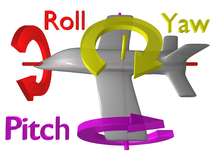The principal axes of rotation in space

One way of generalising the two dimensional angle of rotation is to specify three rotation angles, carried out in turn about the three principal axes. They individually can be labelled yaw, pitch, and roll, but in mathematics are more often known by their mathematical name, Euler angles. They have the advantage of modelling a number of physical systems such as gimbals, and joysticks, so are easily visualised, and are a very compact way of storing a rotation. But they are difficult to use in calculations as even simple operations like combining rotations are expensive to do, and suffer from a form of gimbal lock where the angles cannot be uniquely calculated for certain rotations.

### Euler rotations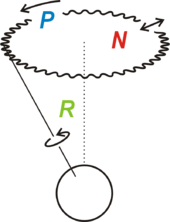Euler rotations of the Earth. Intrinsic (green), Precession (blue) and Nutation (red)

Euler rotations are a set of three rotations defined as the movement obtained by changing one of the Euler angles while leaving the other two constant. Euler rotations are never expressed in terms of the external frame, or in terms of the co-moving rotated body frame, but in a mixture. They constitute a mixed axes of rotation system, where the first angle moves the line of nodes around the external axis z, the second rotates around the line of nodes and the third one is an intrinsic rotation around an axis fixed in the body that moves.

These rotations are called Precession, Nutation, and intrinsic rotation.

### Axis angle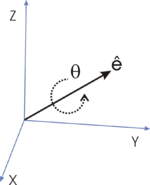A rotation represented by an Euler axis and angle.

A second way of generalising the two dimensional angle of rotation is to specify an angle with the axis about which the rotation takes place. It can be used to model motion constrained by a hinges and Axles, and so is easily visualised, perhaps even more so than Euler angles. There are two ways to represent it;

• as a pair consisting of the angle and a unit vector for the axis, or
• as a vector obtained by multiplying the angle with this unit vector, called the rotation vector.

Usually the angle and axis pair is easier to work with, while the rotation vector is more compact, requiring only three numbers like Euler angles. But like Euler angles it is usually converted to another representation before being used.

### Quaternions

Quaternions are in some ways the least intuitive representation of three dimensional rotations. They are not the three dimensional instance of a general approach, like matrices; nor are they easily related to real world models, like Euler angles or axis angles. But they are more compact than matrices and easier to work with than all other methods, so are often preferred in real world applications.[citation needed]

A rotation quaternion consists of four real numbers, constrained so the length of the quaternion considered as a vector is 1. This constraint limits the degree of freedom of the quaternion to three, as required. It can be thought of as a generalisation of the complex numbers, by e.g. the Cayley–Dickson construction, and generates rotations in a similar way by multiplication. But unlike matrices and complex numbers two multiplications are needed:$\mathbf{x' = qxq^{-1}},$

where q is the quaternion q−1 is its inverse and x is the vector treated as a quaternion. The quaternion can be related to the rotation vector form of the axis angle rotation by the exponential map over the quaternions,$\mathbf{q} = e^{\mathbf{v}/2},$

Where v is the rotation vector treated as a quaternion.

## Four dimensions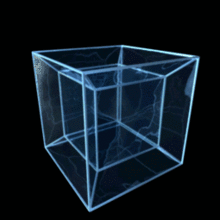An orthogonal projection onto three-dimensions of a hypercube being rotated in four-dimensional Euclidean space.

A general rotation in four dimensions has only one fixed point, the centre of rotation, and no axis of rotation. Instead the rotation has two mutually orthogonal planes of rotation, each of which is fixed in the sense that points in each plane stay within the planes. The rotation has two angles of rotation, one for each plane of rotation, through which points in the planes rotate. If these are ω1 and ω2 then all points not in the planes rotate through an angle between ω1 and ω2.

If ω1 = ω2 the rotation is a double rotation and all points rotate through the same angle so any two orthogonal planes can be taken as the planes of rotation. If one of ω1 and ω2 is zero, one plane is fixed and the rotation is simple. If both ω1 and ω2 are zero the rotation is the identity rotation.

Rotations in four dimensions can be represented by 4th order orthogonal matrices, as a generalisation of the rotation matrix. Quaternions can also be generalised into four dimensions, as even Multivectors of the four dimensional Geometric algebra. A third approach, which only works in four dimensions, is to use a pair of unit quaternions.

Rotations in four dimensions have six degrees of freedom, most easily seen when two unit quaternions are used, as each has three degrees of freedom (they lie on the surface of a 3-sphere) and 2 × 3 = 6.

### Relativity

One application of this is special relativity, as it can be considered to operate in a four dimensional space, spacetime, spanned by three space dimensions and one of time. In special relativity this space is linear and the four dimensional rotations, called Lorentz transformations, have practical physical interpretations.

If a simple rotation is only in the three space dimensions, i.e. about a plane that is entirely in space, then this rotation is the same as a spatial rotation in three dimensions. But a simple rotation about a plane spanned by a space dimension and a time dimension is a "boost", a transformation between two different reference frames, which together with other properties of spacetime determines the relativistic relationship between the frames. The set of these rotations forms the Lorentz group.

## Generalizations

### Orthogonal matrices

The set of all matrices M(v,θ) described above together with the operation of matrix multiplication is called rotation group: SO(3).

More generally, coordinate rotations in any dimension are represented by orthogonal matrices. The set of all orthogonal matrices of the n-th dimension which describe proper rotations (determinant = +1), together with the operation of matrix multiplication, forms the special orthogonal group: SO(n).

Orthogonal matrices have real elements. The analogous complex-valued matrices are the unitary matrices. The set of all unitary matrices in a given dimension n forms a unitary group of degree n, U(n); and the subgroup of U(n) representing proper rotations forms a special unitary group of degree n, SU(n). The elements of SU(2) are used in quantum mechanics to rotate spin.

## Footnotes

1. ^ Lounesto 2001, p.30.
2. ^ Lounesto 2001, pp. 85, 89.
3. ^ Hestenes 1999, pp. 580 - 588.

Wikimedia Foundation. 2010.

### Look at other dictionaries:

• Rotation — This article is about movement of a physical body. For other uses, see Rotation (disambiguation). A polyhedron resembling a sphere rotating around an axis. A rotation is a circular movement of an object around a center (or point) of rotation. A… …   Wikipedia

• Rotation representation (mathematics) — In geometry a rotation representation expresses the orientation of an object (or coordinate frame) relative to a coordinate reference frame. This concept extends to classical mechanics where rotational (or angular) kinematics is the science of… …   Wikipedia

• Rotation (disambiguation) — The term rotation can be used in several ways and includes various topics. Generally, it is used to denote (in 3D) the rotation of movement of a rigid body in such a way that any given point of that body remains at a constant distance from some… …   Wikipedia

• Rotation matrix — In linear algebra, a rotation matrix is a matrix that is used to perform a rotation in Euclidean space. For example the matrix rotates points in the xy Cartesian plane counterclockwise through an angle θ about the origin of the Cartesian… …   Wikipedia

• Mathematics and Physical Sciences — ▪ 2003 Introduction Mathematics       Mathematics in 2002 was marked by two discoveries in number theory. The first may have practical implications; the second satisfied a 150 year old curiosity.       Computer scientist Manindra Agrawal of the… …   Universalium

• Rotation system — In combinatorial mathematics, rotation systems encode embeddings of graphs onto orientable surfaces, by describing the circular ordering of a graph s edges around each vertex.A more formal definition of a rotation system involves pairs of… …   Wikipedia

• mathematics — /math euh mat iks/, n. 1. (used with a sing. v.) the systematic treatment of magnitude, relationships between figures and forms, and relations between quantities expressed symbolically. 2. (used with a sing. or pl. v.) mathematical procedures,… …   Universalium

• Rotation group — This article is about rotations in three dimensional Euclidean space. For rotations in four dimensional Euclidean space, see SO(4). For rotations in higher dimensions, see orthogonal group. In mechanics and geometry, the rotation group is the… …   Wikipedia

• Rotation number — This article is about the rotation number, which is sometimes called the map winding number or simply winding number. There is another meaning for winding number, which appears in complex analysis. In mathematics, the rotation number is an… …   Wikipedia

• Mathematics of Sudoku — The class of Sudoku puzzles consists of a partially completed row column grid of cells partitioned into N regions each of size N cells, to be filled in using a prescribed set of N distinct symbols (typically the numbers {1, ..., N}), so that each …   Wikipedia